# Units of angular measurement Degrees Radians Revolutions Tangential

• Slides: 28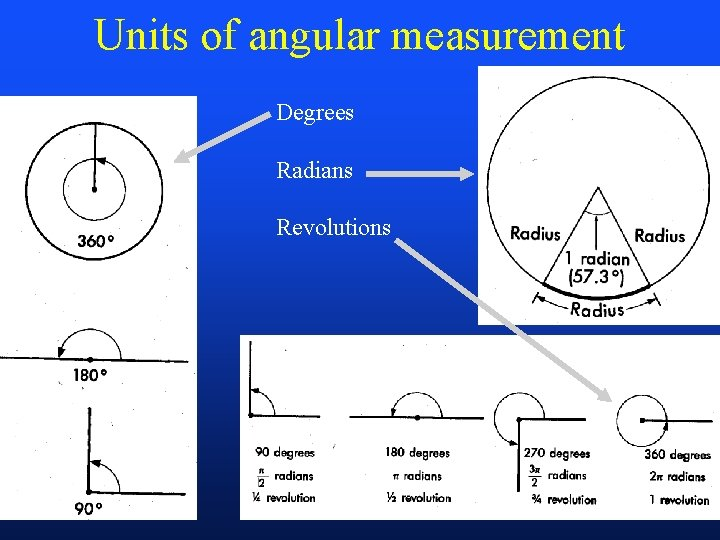Units of angular measurement Degrees Radians Revolutions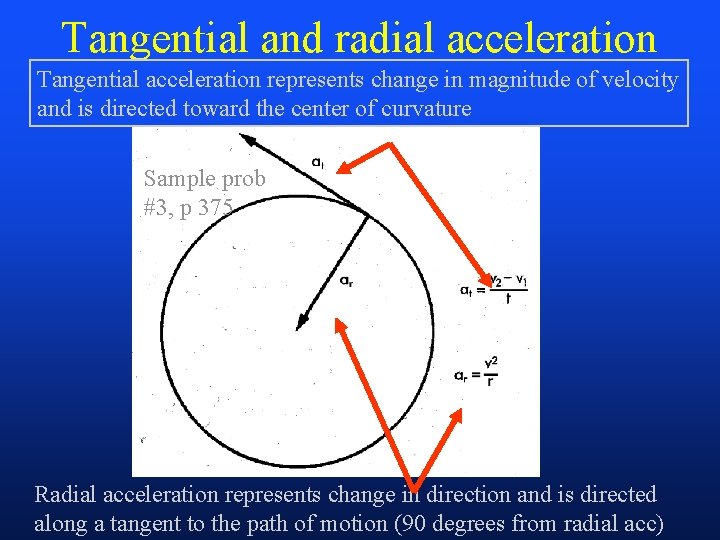Tangential and radial acceleration Tangential acceleration represents change in magnitude of velocity and is directed toward the center of curvature Sample prob #3, p 375 Radial acceleration represents change in direction and is directed along a tangent to the path of motion (90 degrees from radial acc)Linear Kinetics Objectives • Identify Newton’s laws of motion and gravitation and describe practical illustrations of the laws • Explain what factors affect friction and discuss the role of friction in daily activities and sports • Define impulse and momentum and explain the relationship between them • Explain what factors govern the outcome of a collision between two bodies • Discuss the interrelationship among mechanical work, power, and energy • Solve quantitative problems related to kinetic concepts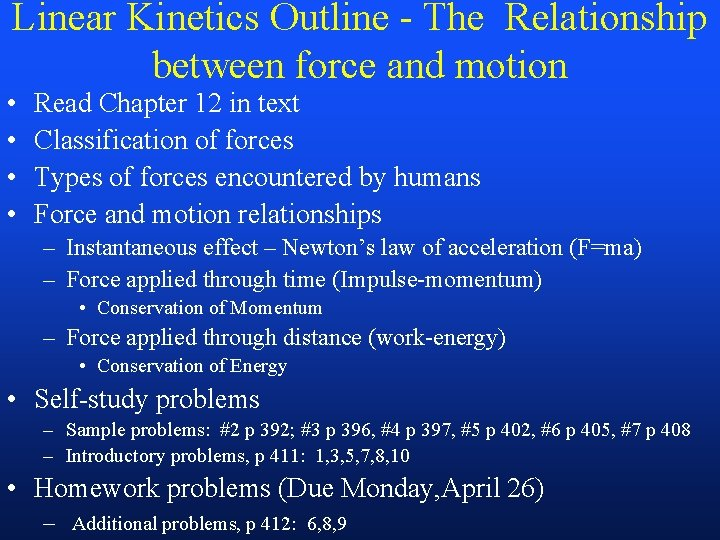Linear Kinetics Outline - The Relationship between force and motion • • Read Chapter 12 in text Classification of forces Types of forces encountered by humans Force and motion relationships – Instantaneous effect – Newton’s law of acceleration (F=ma) – Force applied through time (Impulse-momentum) • Conservation of Momentum – Force applied through distance (work-energy) • Conservation of Energy • Self-study problems – Sample problems: #2 p 392; #3 p 396, #4 p 397, #5 p 402, #6 p 405, #7 p 408 – Introductory problems, p 411: 1, 3, 5, 7, 8, 10 • Homework problems (Due Monday, April 26) – Additional problems, p 412: 6, 8, 9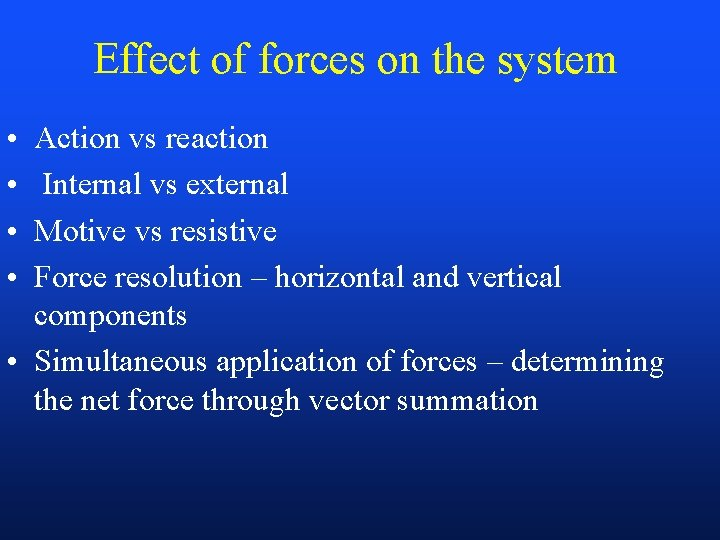Effect of forces on the system • • Action vs reaction Internal vs external Motive vs resistive Force resolution – horizontal and vertical components • Simultaneous application of forces – determining the net force through vector summationExternal forces commonly encountered by humans • Gravitational force (weight = mg) • Ground Reaction Force (GRF)(Figure 12 -4, p 386) – Vertical – Horizontal (frictional) • Frictional force (coefficient of friction) (pp 389 -395) • Elastic force (coefficient of restitution) (pp 399 -402) • Free body diagram - force graph (p 63)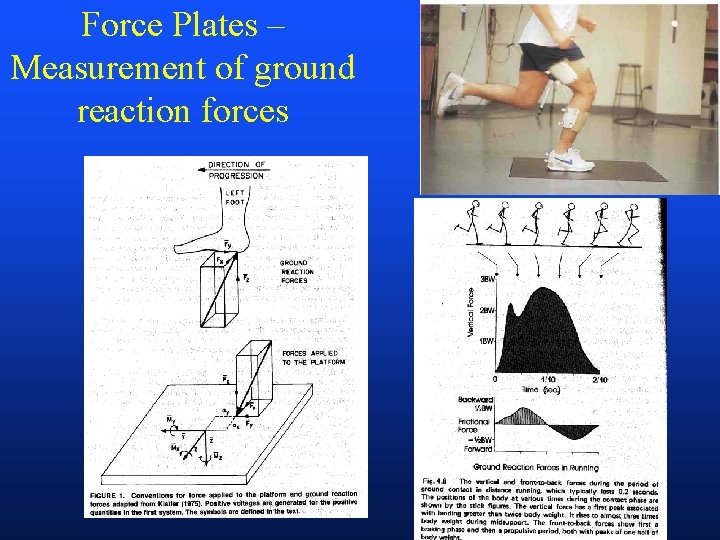Force Plates – Measurement of ground reaction forces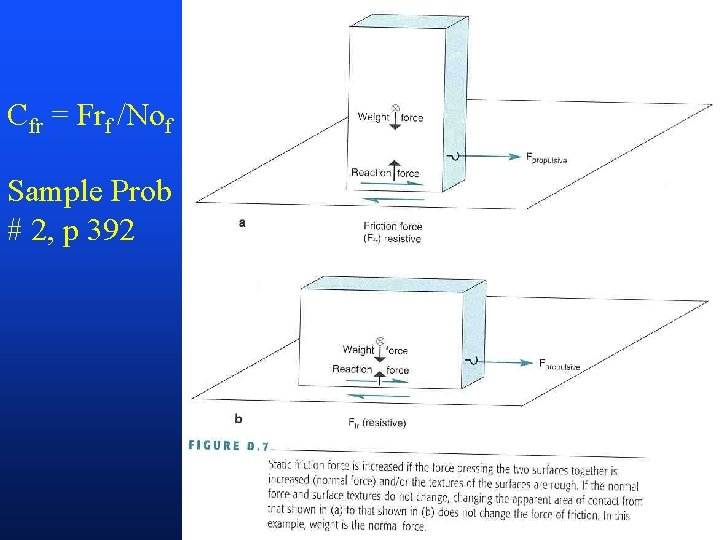Cfr = Frf /Nof Sample Prob # 2, p 392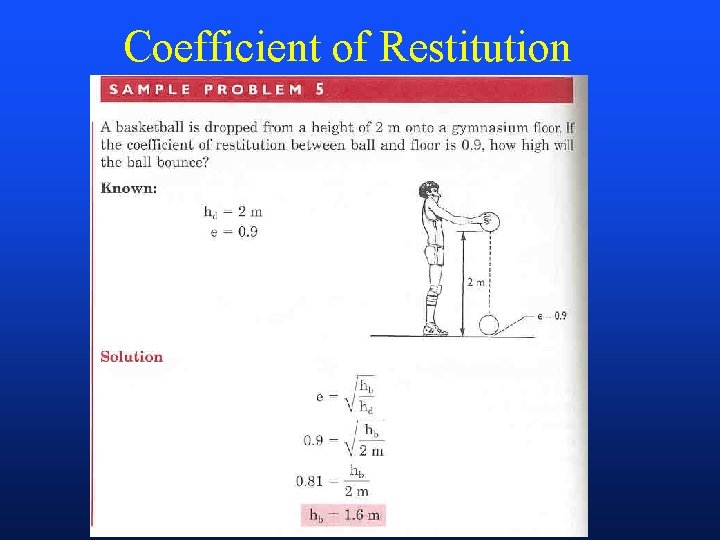Coefficient of Restitution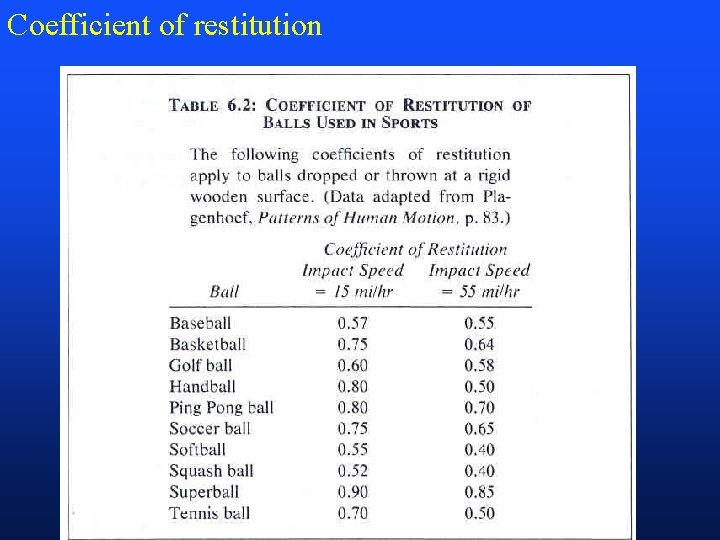Coefficient of restitution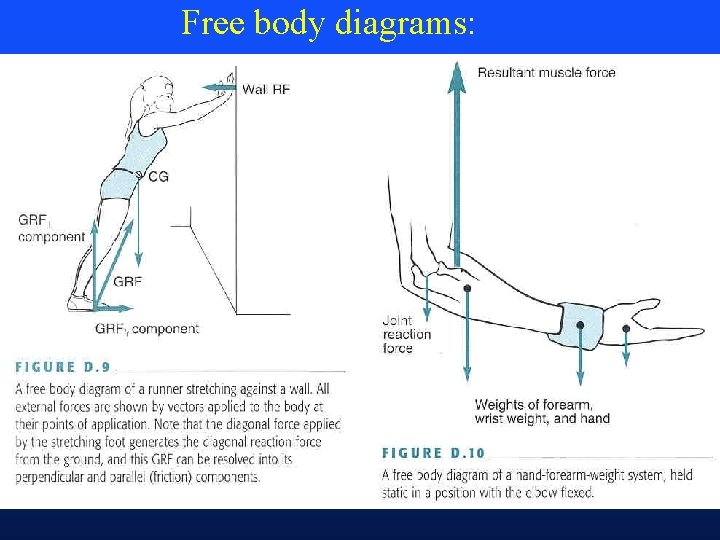Free body diagrams: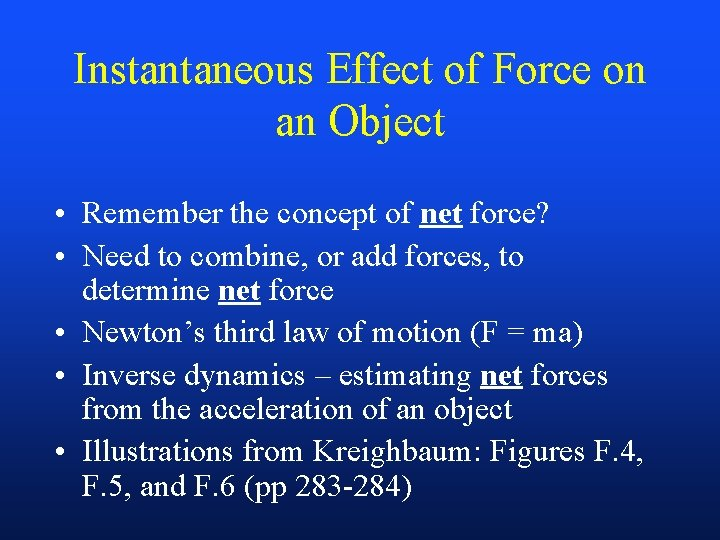Instantaneous Effect of Force on an Object • Remember the concept of net force? • Need to combine, or add forces, to determine net force • Newton’s third law of motion (F = ma) • Inverse dynamics – estimating net forces from the acceleration of an object • Illustrations from Kreighbaum: Figures F. 4, F. 5, and F. 6 (pp 283 -284)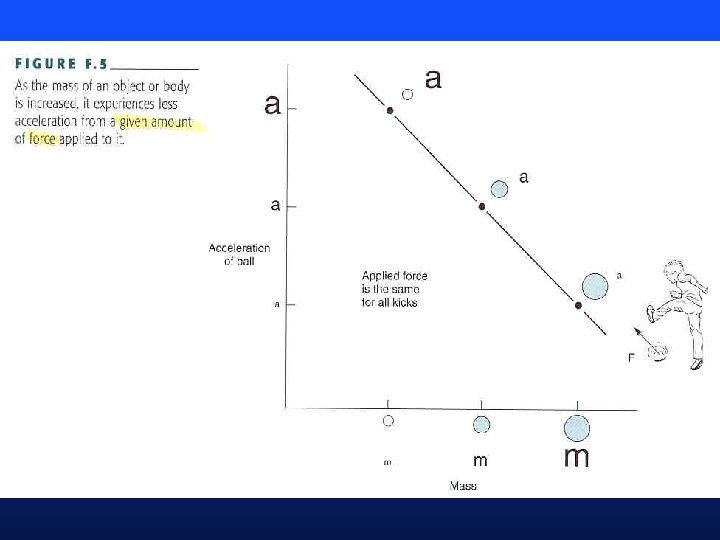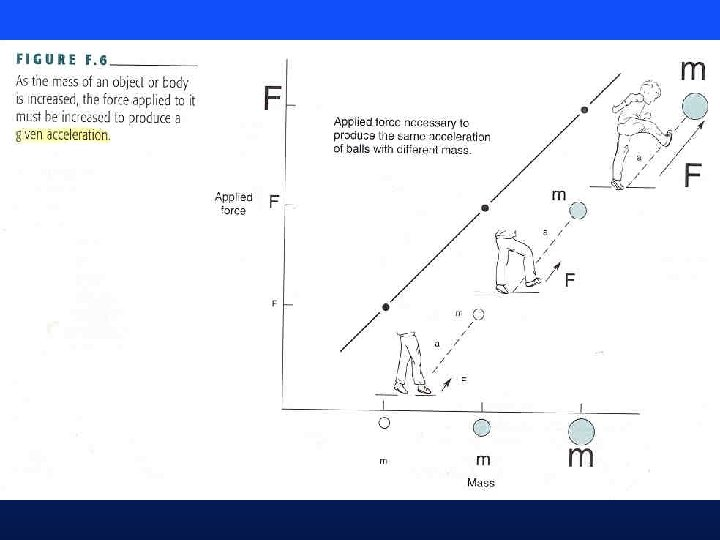Force Applied Through a Time: Impulse. Momentum Relationship (pp 295 -399) • • Force applied through a time Impulse - the area under the force-time curve Momentum - total amount of movement (mass x velocity) An impulse applied to an object will cause a change in its momentum (Ft = mv) • Conservation of momentum (collisions, or impacts) – in a closed system, momentum will not change – what is a closed system?Impulse: area under forcetime curve Impulse produces a change in momentum (m. V) Sample problem #4, p 397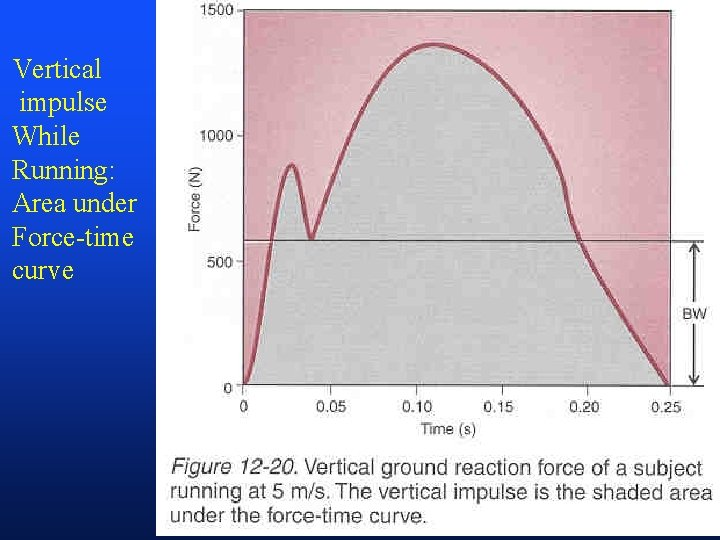Vertical impulse While Running: Area under Force-time curveAnterioposterior (frictional) component of GRF: impulse Is area under Force-time curve Positive and Negative impulse Are equal if Horizontal comp Of velocity is constantConservation of momentum: when net impulse is zero (i. e. the system is closed), momentum does not change Sample prob #3, p 396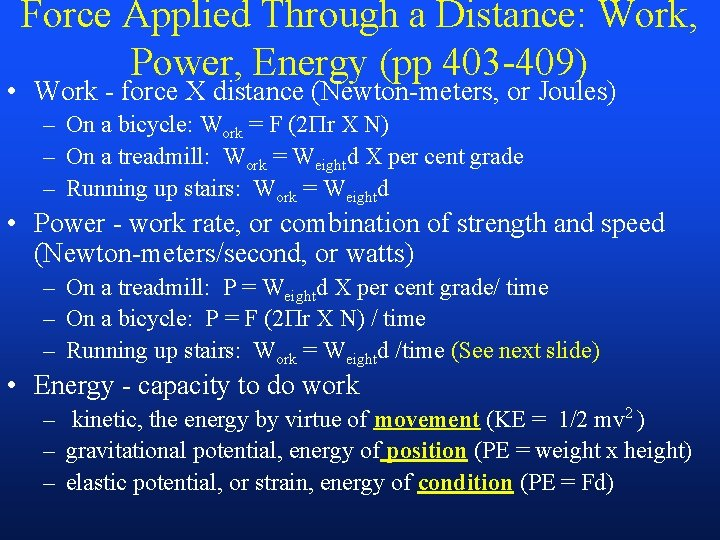Force Applied Through a Distance: Work, Power, Energy (pp 403 -409) • Work - force X distance (Newton-meters, or Joules) – On a bicycle: Work = F (2 r X N) – On a treadmill: Work = Weightd X per cent grade – Running up stairs: Work = Weightd • Power - work rate, or combination of strength and speed (Newton-meters/second, or watts) – On a treadmill: P = Weightd X per cent grade/ time – On a bicycle: P = F (2 r X N) / time – Running up stairs: Work = Weightd /time (See next slide) • Energy - capacity to do work – kinetic, the energy by virtue of movement (KE = 1/2 mv 2 ) – gravitational potential, energy of position (PE = weight x height) – elastic potential, or strain, energy of condition (PE = Fd)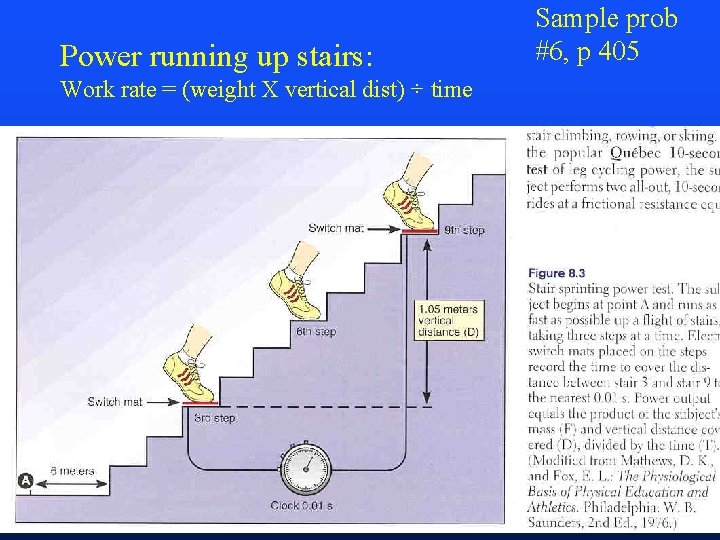Power running up stairs: Work rate = (weight X vertical dist) ÷ time Sample prob #6, p 405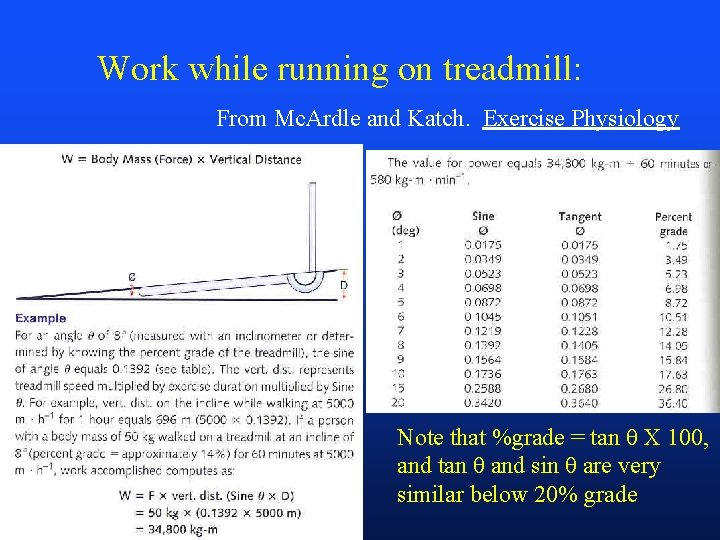Work while running on treadmill: From Mc. Ardle and Katch. Exercise Physiology Note that %grade = tan θ X 100, and tan θ and sin θ are very similar below 20% gradeHomework: Calculating Power on a Treadmill • Problem: What is workload (power) of a 100 kg man running on a treadmill at 10% grade at 4 m/s? • Solution: – Power = force x velocity – Force is simply body weight, or 100 x 9. 8 = 980 N – Velocity is vertical velocity, or rate of climbing • Rate of climbing = treadmill speed x percent grade = 4 m/s x. 1 =. 4 m/s – Workload, workrate, or power = 980 N X. 4 m/s = 392 Watts • Note: 4 m/s = 9 mph, or a 6 min, 40 sec mile • Calculate your workload if you are running on a treadmill set at 5% grade and 5 m/s. – Answer for 200 lb wt (91 kg) is: 223 Watts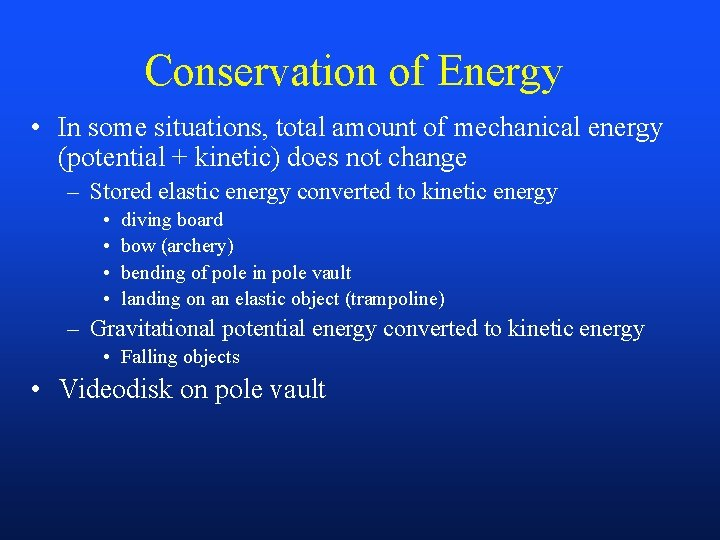Conservation of Energy • In some situations, total amount of mechanical energy (potential + kinetic) does not change – Stored elastic energy converted to kinetic energy • • diving board bow (archery) bending of pole in pole vault landing on an elastic object (trampoline) – Gravitational potential energy converted to kinetic energy • Falling objects • Videodisk on pole vaultEnergy conservation – Case I : elastic potential (strain) and kinetic Potential energy (FD) + Kinetic energy (1/2 mv 2) remains constant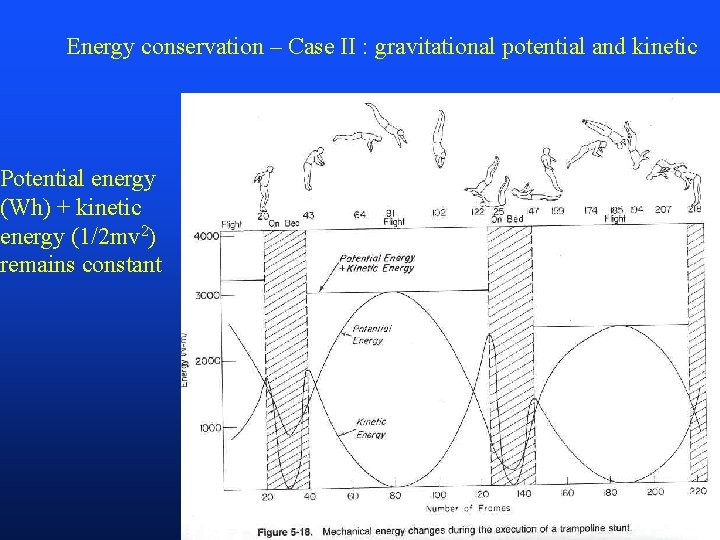Energy conservation – Case II : gravitational potential and kinetic Potential energy (Wh) + kinetic energy (1/2 mv 2) remains constant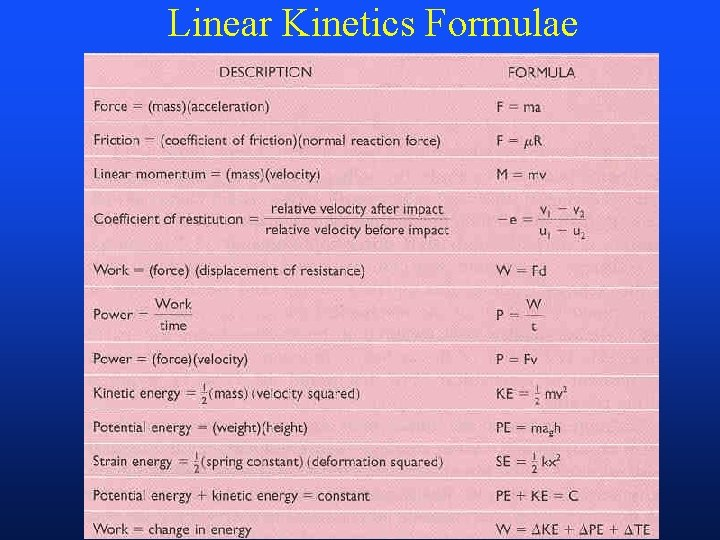Linear Kinetics Formulae